# [PYTHON] Can Machine Learning Predict Parallelograms? (1) Can it be extrapolated?

## Can machine learning be extrapolated?

Hyogo Prefectural Materials Informatics Lecture (4th) Lecture 2 "Descriptor Design Method" at Hyogo Prefectural University Advanced Industry Professor Fujii of the Institute of Science and Technology gave a lecture on descriptor design. I still don't understand the rank drop, but I think it was a very good lecture. I learned a lot.

There was an example of a triangle in the middle of the lecture, and I thought it was true, so I played with a parallelogram as an example.

### Question: Can the area of a parallelogram be predicted given the three features of the length of the two sides and the angle between the two sides? Is extrapolation possible?

First, in order to obtain the area of the parallelogram of the next figure, 1000 random lengths of two sides and angles between the two sides were created. The length of the side is between 100 and 1000, and the angle is 90 degrees or less.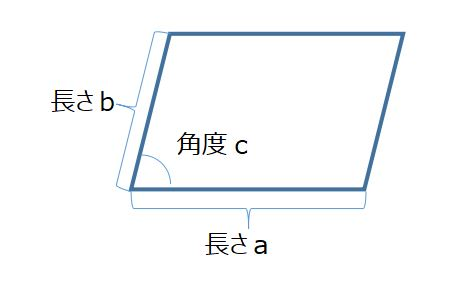If you think about high school mathematics, you know that the formula for the area of a parallelogram can be obtained by multiplying the base and the height, but since you do not know the height, you can find the height using trigonometric functions.

height = b*sin(c)


Once you have the height, multiply it by the bottom.

\begin{align}
area &= height*a\\
&=b*sin(c)*a
\end{align}


I wrote it in a big way, but it's easy because it's a high school math level. Now we have a dataset of three features (length a, b, angle c) and the area of the objective variable.

Here's the question. Question 1. Can parallelograms be machine-learned? What is the accuracy? Question 2. Is extrapolation possible from the results of machine learning? Is it possible to predict given a value other than the one calculated by learning the length of the side?

Of course, Question 2 should not be extrapolated because it is machine learning, but I did not know what it would look like, so I calculated it. Is it possible to extrapolate a parallelogram?

#### Question 1. Can parallelograms be machine-learned? What is the accuracy?

I tried using three machine learnings. ・ Lasso regression ・ Random forest ·neural network Both use scikit-learn. LASSO is used because the feature amount is increased later in the descriptor design and the feature amount is selected and played, so the feature amount is small, but it is calculated by Lasoo. By the way, I calculated Lasso's α as 1 and the hidden layer of neural network (MLP) as 100.

The result. The coefficient of determination looks like this.

Coefficient of determination Learning test
Lasso regression 0.796 0.778
Random forest 0.998 0.989
neural network 0.919 0.913

Looking at this, Random Forest looks good, then neural networks, but what about the graph?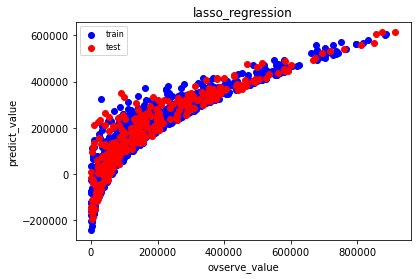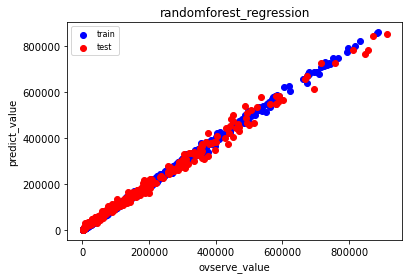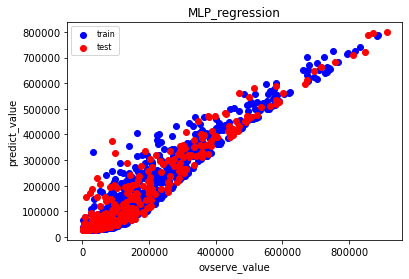Random forest was pretty predictable. Neural networks (MLPs) are also a bit widespread, but they are also well predictable. In the Lasso regression, the larger the number, the better the prediction, but the smaller the number, the wider the prediction.

#### Question 2. Is extrapolation possible from the results of machine learning? Is it possible to predict given a value other than the one calculated by learning the length of the side?

Is it possible to predict the small and large areas of numerical values using this learner?

Angle c
For learning 100～1000 100～1000 0～90
For extrapolation lower side examination 10～90 500 45
For extrapolation upper limit examination 1010～2000 500 45

What will happen with this? b and c are interpolation, and only a is extrapolation. Will it be possible if there is only one?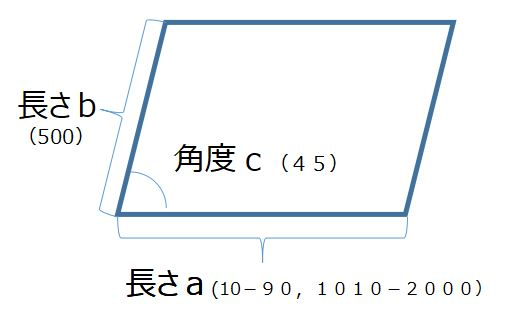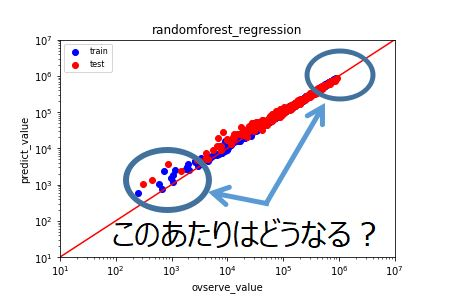It is a graph of the calculation result. As expected? Unexpected?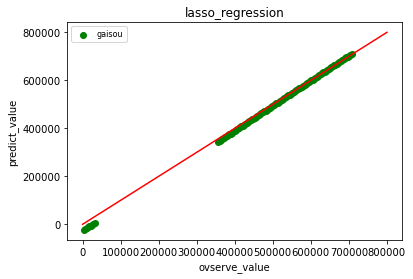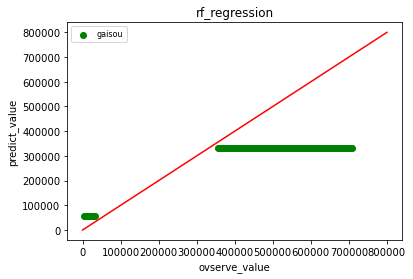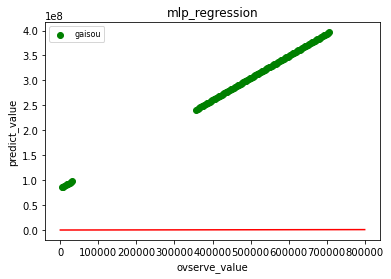The red line is the diagonal, but neither the random forest nor the neural network can be predicted at all with a little extrapolation. Neural networks have irrelevant numbers. It didn't work. The Lasso regression of linear regression is good at predicting extrapolation.

You have to be very careful about extrapolation when making numerical predictions. Please note that only one of the three is out of the learning features, and other than linear regression, the result will be like this.

### If you want to extrapolate even a little, use extrapolation with linear regression.

That's all for today, but what about extrapolation, which looks like interpolation?

#### Question 3: Is it possible to learn with small and large values and make predictions in the meantime?

If you imagine, this is also unpredictable except for linear regression, I'm sure.

So this parallelogram edge continues.